# Merge Sort

Updated:

⚠️ This post was created when I was in high school and is no longer maintained.

we solve a problem recursively, applying three steps at each level of the recursion:

• Divide the problem into a number of subproblems that are smaller instances of the same problem.
• Conquer the subproblems by solving them recursively. If the subproblem sizes are small enough, however, just solve the subproblems in a straightforward manner (using base case).

## 1. Pseudocode

algorithm MergeSort(array, start, end):
if start < end then --> when start == end, this will remain 1 element, so we do not do the last trivial step which sorts the single element.
mid := (start + end) / 2
MergeSort(array, start, mid)
MergeSort(array, mid+1, end)

Merge(array, start, mid, end)

function Merge(array, start, mid, end):
len_l := mid - start + 1
len_r := end - mid --> exclude array[mid]

-- copy two sub-array --
let array_left and array_right be new arrays

For i = 1 to len_l do
array_left[i] = array[start+i-1]
For j = 1 to len_r do
array_right[j] = array[mid+j]

-- set sentinel values --
array_left[i+1]  = ∞
array_right[j+1] = ∞

-- merge two sub-arrays into array --
i := 1 --> initial iterators for two arrays
j := 1
For k = start to end
if array_left[i] ≤ array_right[j] then
array[k] = array_letf[i]
i += 1
else
array[k] = array_right[j]
j += 1



### tips:

1. The shorter array will stop updating its iterator when it encounters the sentinel value at the end of the array so that the sentinel value will not be copied into A. Then, append the remain values of another array to the end of the A;
2. Another sentinel value will not be copied into A as well because k is from start to end rather than (end+1))

## 2. Time complexity

### (1) recurrence equations

It takes time T(n/b) to solve one subproblem of size n=b, and so it takes time aT(n/b) to solve a of them. If we take D(n) time to divide the problem into subproblems and C(n) time to combine the solutions to the subproblems into the solution to the original problem”       —— CLRS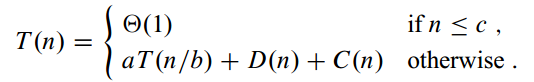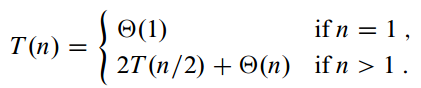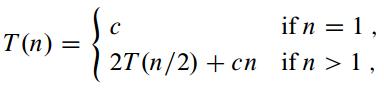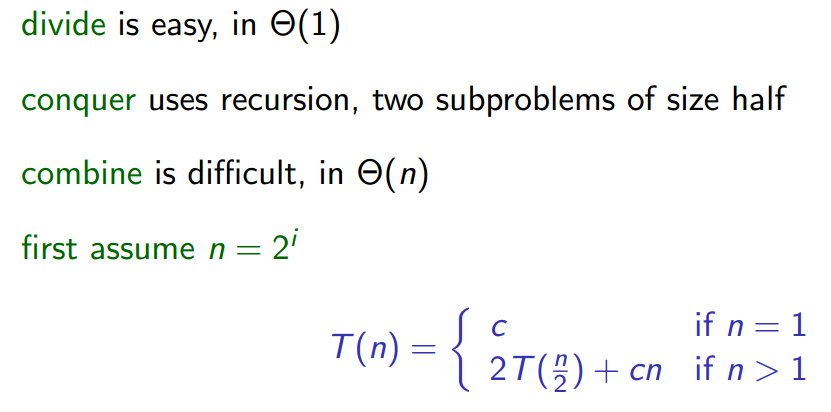### (2) recursion tree

$T(n)$

1. we expand the T(n) into an equivalent tree representing the recurrence: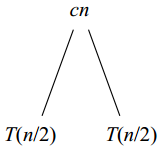Tips: 1).

“The cn term is the root (the cost incurred at the top level of recursion), and the two subtrees of the root are the two smaller recurrences T(n/2).”

2). this represents an addition: $T(n) = cd + T(n/2) + T(n/2)$

1. expand further the recurrence T(n/2):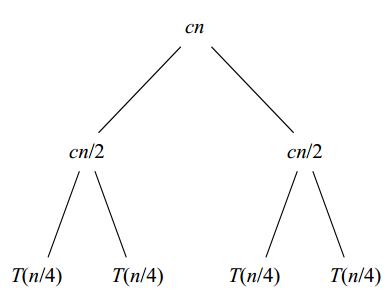2. we continue expanding each node in the tree by breaking it into its constituent parts as determined by the recurrence, until the problem sizes get down to 1: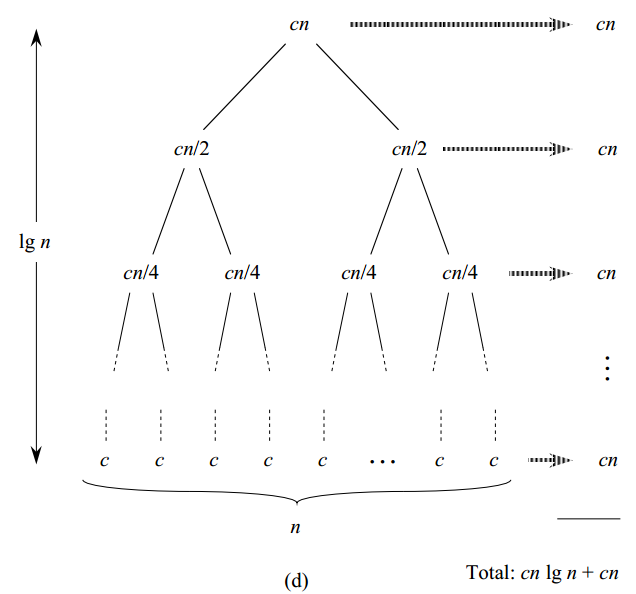Tips: (1) Tree height: $\log_2 n$ (2) Number of levels: $\log_2 n + 1$ (3) Number of leaves: $n\\ (2^i \rightarrow i:level)$ (induction TCRC p58) (4) Total cost: $cn(\log_2n + 1) = cn\log_2n + cn \\ => \Theta(n\log_2n)$

Remark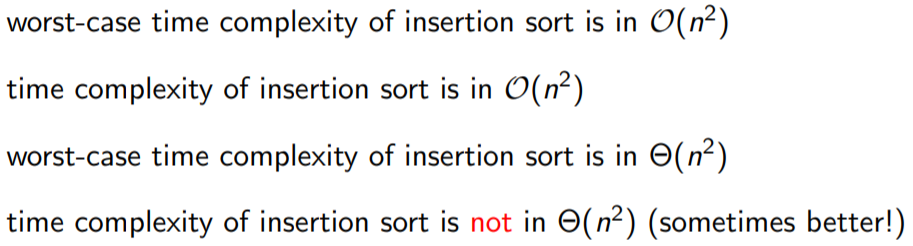Expanation: For example, the time complexity of insertion sort: T(n) == O(n^2^) not Theta(n^2^) because the best-case O(n) is not in the Theta(n^2^) set.

## 4. Code

// Scala
object MergeSortAlgo {
def Merge(array: Array[Double], start: Int, mid: Int, end: Int): Unit = {
var len_l = mid - start + 1
var len_r = end - mid

var i, j = 0
var array_left  = new Array[Double](len_l)
var array_right = new Array[Double](len_r)
for (i <- 0 until len_l) {
array_left(i) = array(start+i)
}
for (j <- 0 until len_r) {
array_right(j) = array(mid+j+1)
}

array_left  :+= Double.MaxValue
array_right :+= Double.MaxValue

i = 0
j = 0
var k = 0
for (k <- start to end) {
// printf("start: %d, end: %d, i: %d, j: %d, k: %d\n", start, end, i, j, k)
if (array_left(i) <= array_right(j)) {
array(k) = array_left(i)
i += 1
} else {
array(k) = array_right(j)
j += 1
}
}
}

def MergeSort(array: Array[Double], start: Int, end: Int): Array[Double] = {
if (start < end) {
var mid = (start + end) / 2

MergeSort(array, start, mid)
MergeSort(array, mid+1, end)

Merge(array, start, mid, end)
}
return array
}

def main(args: Array[String]): Unit = {
var array = Array(9999, 4234,234234,23,234,-134,4123,41,578,-342,55,0.2323,0.325)
println("\nBefore sorting:")
println(array.deep.mkString(" | "))
println("\nAfter sorting:")
println(MergeSort(array, 0, array.length-1).deep.mkString(" | "))
}
}


# Python
import sys

def MergeSort(array, start, end):
if start < end:
mid = (start + end) // 2

MergeSort(array, start, mid)
MergeSort(array, mid+1, end)

Merge(array, start, mid, end)

def Merge(array, start, mid, end):
array_left = array[start: mid+1]
array_right = array[mid+1: end+1]

inf = sys.maxsize
array_left.append(inf)
array_right.append(inf)

i, j = 0, 0
for k in range(start, end+1):
if array_left[i] <= array_right[j]:
array[k] = array_left[i]
i += 1
else:
array[k] = array_right[j]
j += 1

if __name__ == "__main__":
array = [4234,234234,23,234,-134,123,41,578,-342,55,0.9323,0.325]
end = len(array) - 1

print("Before sorting: ", array)
MergeSort(array, 0, end)
print("After sorting:  ", array)



### tips:

The stop condition in MergeSort() cannot be “start <= end” (partition the array into [X] and [Ø]), because this will causes infinite loop. (之后二分的结果会一直为0 -> 0 == 0)

Tags: